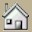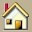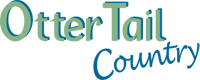CabinsThe LodgeRatesBoats & LiftsResort LayoutAvailability July 2018 August 2018Contact UsPoliciesTheLakeDirectionsHistoryResort Photos The Lodge on Otter Tail Lake 28525 Cty Hwy 145 Battle Lake, MN  56515 (218) 495-2468 (800) 334-3679 Vacation@YourResort.com

Check Back for our Availability, it is updated Regularly. BLACK IS UNAVAILABLE.

 July 2018 - S M T W Th F Sa Su M T W Th F Sa Su M T W Th F Sa Su M T W Th F Sa Su M T Units 1 2 3 4 5 6 7 8 9 10 11 12 13 14 15 16 17 18 19 20 21 22 23 24 25 26 27 28 29 30 31 Blue Heron X X X X X X X X X X X X X X X X X X X Cabin 02 X X X X X X X X X X X X X X X X X X X X X X X X X X X Cabin 04 X X X X X X X X X X X X X X X X X X X X X X X X X X Cabin 05 X X X X X X X X X X X X X X X X X X X X X X X X X X X X X X X Cabin 07 X X X X X X X X X X X X X X X X X X X X X X X Cabin 08 X X X X X X X X X X X X X X X X X X X X X X X X X X X X X X X Cabin 09 X X X X X X X X X X X X X X X X X X X X X X X X X X X Cabin 10 X X X X X X X X X X X X X X X X X X X X X X X X X X X Cabin 11 X X X X X X X X X X X X X X X X X X X X X X X X X X X X Cabin 12 X X X X X X X X X X X X X X X X X X X X X X X X X X Cabin 13 X X X X X X X X X X X X X X X X X X X X X X X Cabin 14 X X X X X X X X X X X X X X X X X X X X X X X
In fact, handbags replica knows that the high imitation gucci replica handbags is very deep, mixed with fish and dragons, and there are many hermes replica handbags. It may be replica handbags of styles ranging from a few hundred to hermes replica .

Otter Tail Country Tourism Association
If the specific date you are looking for is not available. Contact us and we can put you on a waiting list or go the Otter Tail Country website. It lists all resorts in the area and can help you find one with openings.
Tell them that The Lodge on Otter Tail Lake sent you.